# Logik game

Letter game Logik is a two player game, which has the following rules:
1. The first player thinks five-letter word in which no letter is not
repeated.
2. The second player writes a five-letter word.
3. The first player answers two numbers - the first number indicates how many letters written word coincides with the imaginary word, and is also on the correct position; the second number indicates how many letters written word is contained in the imaginary word, but is not the right place.
4. Steps 2 and 3 are repeated until the second player imaginary word guess.
Recording one game of two friends looked like this:
SONET 1 2
MUDRC 0 2
PLAST 0 2
KMOTR 0 4
ATOLY 1 1
DOGMA 0 2

The next move was meant by the word guessed. Determine which word
it was.

Note:
Our presented solution contains a program in PHP language, which will solve the given combination of letters - by brute force, quickly and painlessly.

Result

x = (Correct answer is: STROM)#### Solution:

x = STROM
```function match(\$s1, \$s2, \$q, \$w)
{
\$a = \$b = 0;
for(\$i=0;\$i<5;\$i++)
{
if(\$s1{\$i} == \$s2{\$i})
{
\$a++;
}
else
{
\$b += strpos(\$s2, \$s1{\$i})!==false;
}
}

return \$a == \$q && \$b == \$w;
}

for(\$a=65; \$a<91; \$a++)
{
for(\$b=65; \$b<91; \$b++)
{
for(\$c=65; \$c<91; \$c++)
{
for(\$d=65; \$d<91; \$d++)
{
for(\$e=65; \$e<91; \$e++)
{
\$s=chr(\$a).chr(\$b).chr(\$c).chr(\$d).chr(\$e);
if(!match(\$s,"SONET", 1, 2)) continue;
if(!match(\$s,"MUDRC", 0, 2)) continue;
if(!match(\$s,"PLAST", 0, 2)) continue;
if(!match(\$s,"KMOTR", 0, 4)) continue;
if(!match(\$s,"ATOLY", 1, 1)) continue;
if(!match(\$s,"DOGMA", 0, 2)) continue;

echo \$s;
exit;
}
}
}

}
}
```

Leave us a comment of example and its solution (i.e. if it is still somewhat unclear...):

Showing 0 comments:Be the first to comment!#### To solve this example are needed these knowledge from mathematics:

See also our permutations calculator. Would you like to compute count of combinations?

## Next similar examples:

1. Hockey players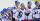After we cycle five hockey players sit down. What is the probability that the two best scorers of this crew will sit next to each other?
2. NumbersHow many different 7 digit natural numbers in which no digit is repeated, can be composed from digits 0,1,2,3,4,5,6?
3. Playing cards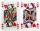How many possible ways are to shuffle 7 playing cards?
4. 7 heroes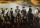9 heroes galloping on 9 horses behind. How many ways can sort them behind?
5. Flags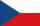How many different flags can be made from colors white, red, green, purple, orange, yellow, blue so that each flag consisted of three different colors?
6. Vans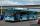In how many ways can 9 shuttle vans line up at the airport?
7. Seating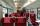How many ways can 6 people sit on 3 numbered chairs (e. G. , seat reservation on the train)?
8. WordWhat is the probability that a random word composed of chars T, H, A, M will be the MATH?
9. Shelf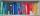How many ways are there to arrange 6 books on a shelf?
10. Phone numbersHow many 7-digit telephone numbers can be compiled from the digits 0,1,2,..,8,9 that no digit is repeated?
11. RectanglesHow many rectangles with area 3032 cm2 whose sides is natural numbers are?
12. Pairs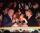At the table sit 8 people, 4 on one side and 4 on the other side. Among them are 3 pairs. Every pair wants to sit opposite each other. How many ways can they sit?
13. Lock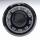Combination lock will open when the right choice of 5 numbers (from 1 to 12 inclusive) is selected. A. How many different lock combinations are possible? B. Is he combination lock named appropriately?
14. Subsets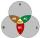How many are all subsets of set ??
15. Kids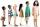How many different ways can sit 8 boys and 3 girls in line, if girls want to sit on the edge?
16. School tripThe class has 19 students. What different ways students can be accommodated in the hostel, where available 3× 2-bed, 3× 3-bed and 1× 4-bed rooms. (Each room has its unique number)
17. Toys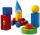3 children pulled 12 different toys from a box. Many ways can be divided toys so that each children had at least one toy?